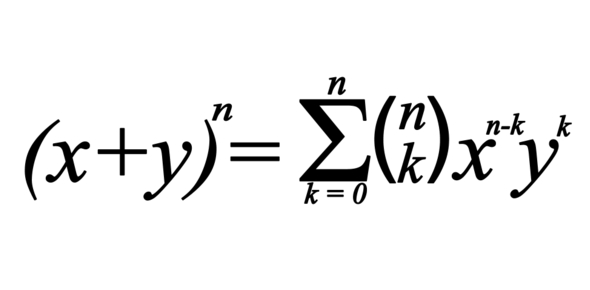# Binomial Theorem Quiz

12 Questions | Attempts: 1842
ShareSettingsThe Binomial Theorem is a quick way of expanding a binomial expression that has been raised to some power. Most students have issues when it comes to working out these problems, having some practice helps. Take up the Binomial theorem quiz below to sharpen your math skills. All the best!

• 1.
The binomial expansion of (1-b)^  is the same as that of (1+b)^n except that the coefficients have alternate + and - signs.
• A.

True

• B.

False

• 2.
In the expansion of (x^3-2/x^2)^10, find the coefficient of 1/x^5.
• A.

13440

• B.

3360

• C.

-15360

• 3.
There is no difference between the power n and the expansion of the binomial theorem.
• A.

True

• B.

False

• 4.
Find the term x^3 in the expansion of (1+5)^2(1-2x)^6. 150x^3
• A.

150x^3

• B.

140x^3

• C.

91x^3

• 5.
In the expansion of (2+3x)^n, the coefficients of x^3 and x^4 are in the ratio of 8:15. Find the value of n.
• A.

10

• B.

8

• C.

3

• 6.
Who created the special triangle?
• 7.
Find the 7th term of (2+x)^10.
• A.

3360x^6

• B.

3000x^6

• C.

4500x^7

• 8.
BINOMIAL , in mathematics, a word first introduced by Robert Recorde (1557) to denote a quantity composed of the sum or difference to two terms; as a+b, a-b.
• A.

True

• B.

False

• 9.
Find the coefficient of x^6 in (1-3x)^8
• A.

20412

• B.

10512

• C.

23110

• 10.
Find the coefficient of x^3 in the expansion of (3-4x)(2-x/2)^7
• A.

435

• B.

-882

• C.

632

• 11.
With the help of this binomial theorem for positive integral index indices , we can expand any power of x + y into a sum of terms forming a polynomial.
• A.

True

• B.

False

• 12.
Find the coefficients of x^2 in the expansion of (1-2x)^5.23
• A.

23

• B.

40

• C.

51

## Related TopicsBack to top
×

Wait!
Here's an interesting quiz for you.